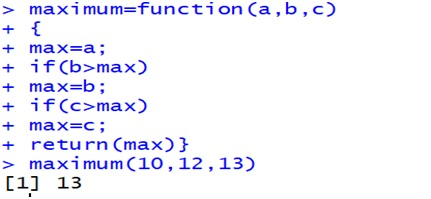# Maximum of 3 numbers in R language using user-defined function

Here, we are creating a user-defined function in R programming language to find the maximum of 3 numbers.
Submitted by Akash Kumar, on November 08, 2018

Aim: To create user-defined function in R to find maximum of three numbers.

Syntax:

To create function in R following syntax is used:

```    Function-name=function(parameter)
{
#body of function
}
```

Where,

• Function-name is the name of user-defined function.
• function is the keyword which is used in R to create function
• #body of function basically defines the property of function.

Code:

```    maximum=function(a,b,c)
{
max=a;
if(b>max)
max=b;
if(c>max)
max=c;
return(max);
}
```

OutputHence, we successfully created function in R which will calculate maximum among three numbers in R.